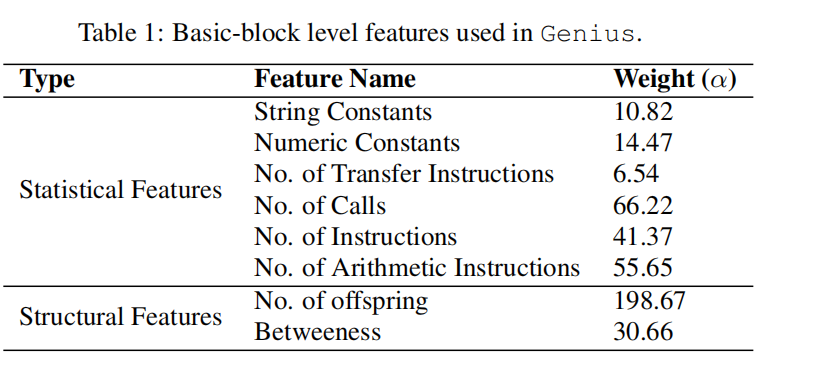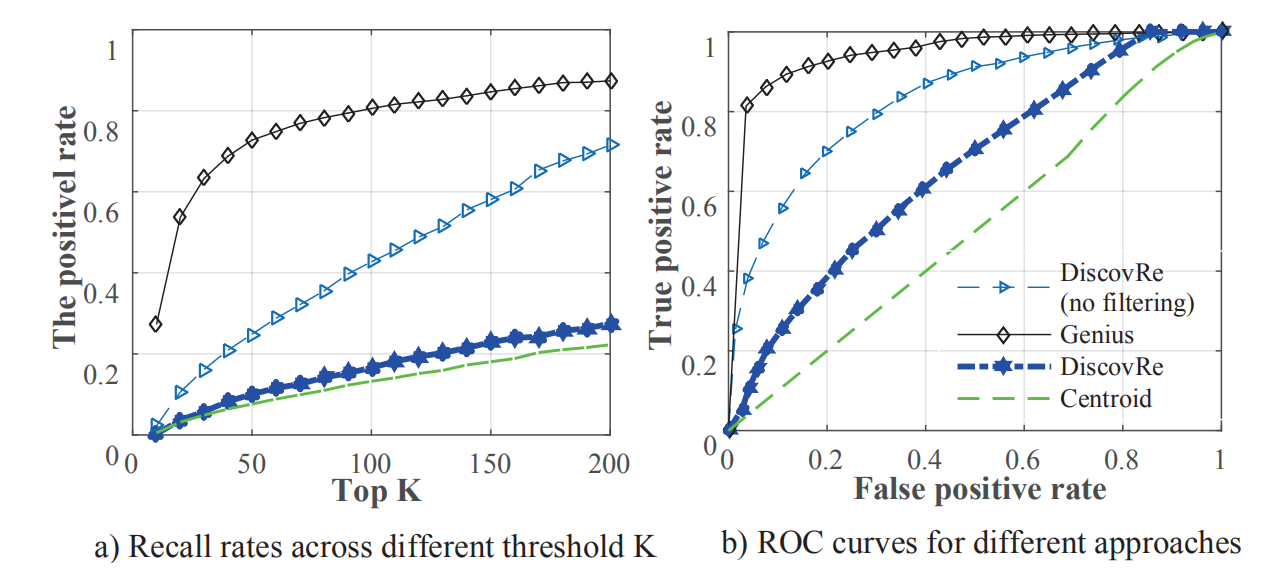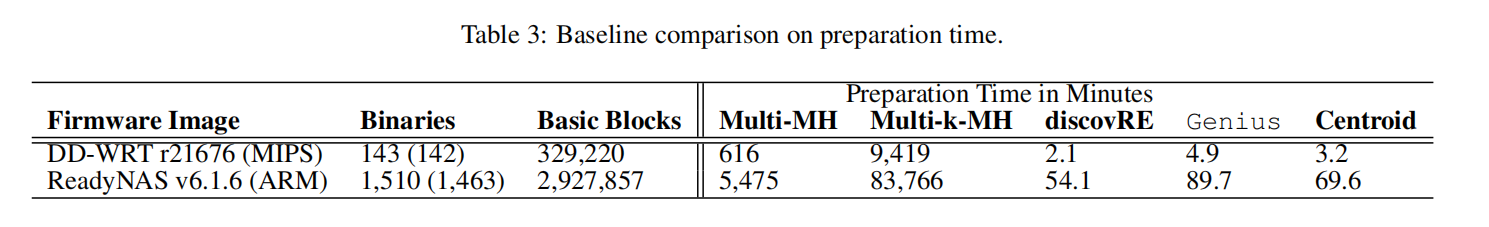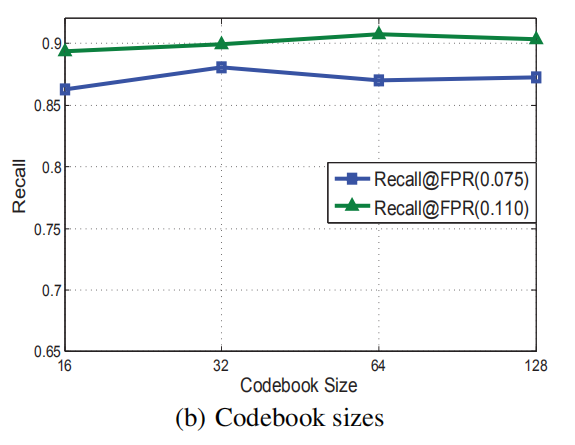# Scalable Graph-based Bug Search for Firmware Images(Genius)阅读笔记

## 二进制代码相似性检测

Posted by Yunlongs on March 20, 2020

## Scalable Graph-based Bug Search for Firmware Images(Genius)

### 属性控制流图

1. no.of offspring为控制流图中孩子节点的数量，它可以帮助我们定位一个节点在图中的第几layer。
2. betweenness centrality可以给出一个节点在图中的中心程度。### 具体实现

#### 1.编码本生成

##### 1.1 原生特征相似性生成

$\operatorname{cost}(v, \hat{v})=\frac{\sum_{i} \alpha_{i}\left|a_{i}-\hat{a}_ {i}\right|}{\sum_{i} \alpha_{i} \max \left(a_{i}, \hat{a}_{i}\right)}$

$\kappa\left(g_{1}, g_{2}\right)=1-\frac{\operatorname{cost}\left(g_{1}, g_{2}\right)}{\max \left(\operatorname{cost}\left(g_{1}, \Phi\right), \operatorname{cost}\left(\Phi, g_{2}\right)\right)}$

#### 2. 特征编码

$N N\left(g_{i}\right)=\arg \max _ {c_{i} \in \mathcal{C}} \kappa\left(g_{i}, c_{j}\right)$

Bag-of-feature encoding.

$q\left(g_{i}\right)=\sum_{g_{i}: N N\left(g_{i}\right)=c_{j}}[\mathbb{1}(1=j), \ldots, \mathbb{1}(n=j)]^{T}$

$q\left(g_{i}\right)=\sum_{g_{i}: N N\left(g_{i}\right)=c_{j}}\left[\mathbb{1}(1=j) \kappa\left(g_{i}, c_{1}\right), \ldots, \mathbb{1}(n=j) \kappa\left(g_{i}, c_{n}\right)\right]^{T}$

(a)为F1在x86和mips下编译得到的控制流图，以及另一个函数F2在mips下编译得到的控制流图。
(b)为经过训练所得到的的编码本。
(c)为使用图匹配算法对(a)中的三个控制流图进行匹配得出的相似性结果，可以看出其预测结果存在较大的偏差。
(d)为VLAD encoding在不同距离度量标准下的相似性分数，可以看出在欧几里德距离下能够区分出相同与不相同的函数。#### 在线搜索

LSH方法可以学习得到一个投影：如果两个点在特征编码空间中距离很近，那么其在hash空间中的投影也很近。给定编码后的特征$q(g)$，我们将投影函数$h_i$定义如下：

$h_{i}(q(g))=\lfloor(\mathbf{v} \cdot q(g)+b) / w\rfloor$

LSH https://blog.csdn.net/guoziqing506/article/details/53019049/ MinHash原理 https://www.jianshu.com/p/535c537a5766

### 实验评估

#### 2.数据集准备

dataset architectures compiler versions optimization levels
BusyBox(v1.21 and v1.20) x86 gcc v4.6.2/v4.8.1 O0-O3
OpenSSL (v1.0.1f and v1.0.1a) ARM clang v3.0
coreutils(v6.5 and v6.7) MIPS

#### 3.跨平台Baseline比较

Evaluation metrics： 使用召回率和假正率来测试我们的baseline方法。例如，对于每个查询q，在总共L个函数中匹配到了m个函数，我们认为top-K个检索到的函数为正例，正确匹配的函数个数为$\mu$为真正例，剩下的$K-\mu$为假正例。所以我们可以计算得到召回率为recall$(q)=\frac{\mu}{m}$，假正率为$F P R(q)=\frac{(K-\mu)}{L-m}$。

Baseline 系统的建立 ：使用三个具有代表性且先进的跨平台bug搜索技术来建立我们的评估baseline：discovRe，Multi-MH and Multi-k-MH ，a centroid based search

Accuracy 比较： 从baseline数据集中随机选取了1000个函数进行查询。从图中可以看出Genius方法相较于baseline方法实现了更高的Accuracy。

Efficiency 比较： 线下预处理比较：#### 4.参数学习

B.编码本大小： 编码本的大小对准确率额影响并不是很大，因此我们倾向与选取小的size来降低预处理的时间。C.训练集大小： 生成编码本所采样的训练集大小，是个很重要的参数，越大准确度越高，但当10w个函数时就已足够。

### Discussion

1. 高度依赖CFG提取的质量，可以考虑比IDA更好的CFG提取工具
2. 内联函数会影响CFG流程。
3. Genius受CFG的大小影响较大，因为越小的CFG月可能发生碰撞